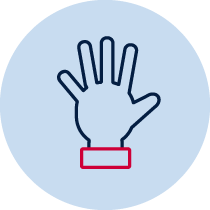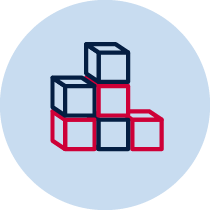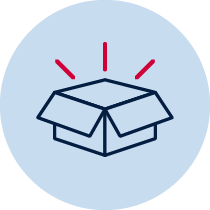# Whole number - tell me all you know about 10Practical Build and make Resource required

## Number – whole number

• describes mathematical situations and methods using everyday and some mathematical language, actions, materials, diagrams and symbols MA1-1WM
• uses objects, diagrams and technology to explore mathematical problems MA1-2WM
• supports conclusions by explaining or demonstrating how answers were obtained MA1-3WM
• applies place value, informally, to count, order, read and represent two- and three-digit numbers MA1-4NA

## Content

Recognise, model, read, write and order numbers to at least 100; locate these numbers on a number line (ACMNA013)

• represent two-digit numbers using objects, pictures, words and numerals

• uses a range of strategies and informal recording methods for addition and subtraction involving one- and two-digit numbers MA1-5NA
• uses a range of mental strategies and concrete materials for multiplication and division MA1-6NA

## National Numeracy Learning Progression mapping to the NSW mathematics syllabus

When working towards the outcome MA1-4NA the sub-elements (and levels) of quantifying numbers (QuN6-QuN8) and understanding money (UnM3-UnM4) describe observable behaviours that can aid teachers in making evidence-based decisions about student development and future learning.

## Teacher instructions

The purpose of this task is to gauge students’ understanding of whole number concepts such as:

• make, name and record numbers
• part-part-whole relationships
• subitising

Teacher will explain to students that they will make a poster with all the different ways the number can be represented. Students may represent the number 10 by modelling, drawing, writing words or numerals.

## Student instructions

Students are given paper to create a poster of all the different ways they can represent 10.

## Where to next?

Students need a deep understanding of the numbers to 10 in terms of their quantity and be able to manipulate into various parts for example 10 is 2 more than 8 or 7 and 3 or 5 and 3 and 2. Focus on combinations and where and how they are useful for addition and subtraction. From this task teachers can determine if students have this deep understanding or if too much emphasis is on the count, symbols, numerals or numbers.